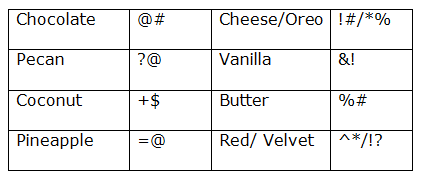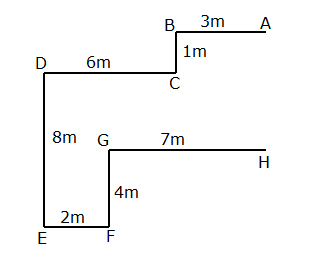# IBPS PO Prelims Reasoning (Day-16)

Dear Aspirants, Our IBPS Guide team is providing new series of Reasoning Questions for IBPS PO Prelims 2020 so the aspirants can practice it on a daily basis. These questions are framed by our skilled experts after understanding your needs thoroughly. Aspirants can practice these new series questions daily to familiarize with the exact exam pattern and make your preparation effective.

Start Quiz

Coding decoding

Direction (1-5): Study the following information carefully and answer the below questions.

In a certain code language,

‘Chocolate Pecan Coconut Pineapple’ means ‘@#  [email protected] [email protected] +\$’,

‘Cheese Chocolate Vanilla Oreo’ means ‘*% &! @# !#’,

‘Pecan Oreo Cheese Butter’ means ‘%# !# *% [email protected]’,

‘Coconut Vanilla Butter Red Velvet’ means ‘!? %# &! +\$ ^*’

1) What is the code for ‘Vanilla’ in the given code language?

A.!#

B.*%

C.&!

E.None of these

2) What is the code for ‘Red Pecan’ in the given code language?

C.%# *%

D.!# ^*

E.Either a or b

3) What does “@#” represent in a code language?

A.Pineapple

B.Coconut

C.Pecan

D.Chocolate

E.None of the above

4) If “Cheese Butter” represents a code “!# %#”, then what does “*% &! +\$” represent in a code language?

A.Coconut butter Red

B.Vanilla Coconut Butter

C.Oreo Vanilla Coconut

D.Pecan Chocolate vanilla

E.None of the above

5) What is the code for ‘Velvet Oreo Pineapple’?

A[email protected] !? ^*

B.!# *% [email protected]

C.!# [email protected] !?

D.^* *% [email protected]

E.Can’t be determined

Inequality

Direction (6-7): In each of the following questions, the relationship between different elements is shown in the statements followed by some conclusions. Find a conclusion which logically follows.

6) Statement:

P ≤ Y ≥ J ; L = U < O; I > L ≤ G = Y

Conclusion:

I). O > I

II). G ≥ J

III). U = Y

A.Only I follows

B.Only II follows

C.Only III follows

D.All follow

E.None follows

7) Statement:

M < S < A < C ;  O ≥ C = T ≥ B; K ≥ L > T > V

Conclusion:

I). K = B

II). A < L

III). K > B

A.Only II follows

B.Only III follows

C.Both II and III follow

D.Only II and either I or III follow

E.None follows

Direction sense

Direction (8-10): Study the following information carefully and answer the questions given below.

A man from point A walks towards west for 3m and turns to his left at point B,from where he walks for 1m and reaches point C.Then, he turns to his right and walks for 6m to reach point D where he turns to his left. Then he walks for 8m and reaches point E. He walks for 2m after taking a left turn and reaches point F. Then he walks for 4m towards the north to reach point G from where he turns to his right and walks for 7m to reach point H.

8) What is the shortest distance between points A and H?

A.6m

B.8m

C.7m

D.5m

E.None of these

9) In what direction is point D with respect to point G?

A.North

B.Southeast

C.Northwest

D.South

E.None of these

10) What is the approximate shortest distance between points E and B?

A.12m

B.11m

C.10m

D.13m

E.None of these

Directions (1-5):Directions (6-7) :

I). O > I –> O > U = L < I –> False

II). G ≥ J –> G = Y ≥ J –>True

III). U = Y –> U =  L ≤ G = Y –> False

Hence, Option B is correct.

I). K = B –> K ≥ L > T ≥ B –>False

II). A < L –> A < C = T < L –>True

III). K > B –> K ≥ L > T ≥ B –>True

Hence, Option C is correct.

Directions (8-10) :